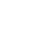Live chatIn this paper, a company in Onondaga County, NY that has a sale value of over \$ 750,000 is evaluated. It is used for the calculation of the Net Operating Income and the various values as anticipated in the exercise. The property selected is in 586 Myrtle Ave, Albany NY 12208. It is 95% occupied. The building offers space for residential purposes. It is a five-stored structure giving accommodation to ten tenants. The total amount in rental income is \$57,000 per annum. The amount accruing as costs are as follows: repairs and maintenance \$ 1,900, insurance \$900, taxes \$800, and other costs like security, cleaning amounts to \$1,200. All these expenses are computed on an annual basis. In the computation of the Net Operating Income, all the income generated by the property is considered against all the expenses incurred due to the property. From the above figures, the total income accruing to the property in a year is \$ 57,000. The total costs are 5,700. The Net Operating Income is therefore the difference in the figures, which is \$51,300.In this section, the Debt Service Coverage Ratio is given as 1:25, the amortization period is 20 years and the interest rate is at 6%. From the above data, the ratio is more than one. This means that the property is in a position to meet its costs. The income it generates is higher than the costs the property incurs in payments of loan charges. That is the principal sum and the interest. The computation of the total debt service amount is arrived at by the use of the Net Operating Income and the ratio (Khan, 1993). By dividing the NOI with the total debt service amount, the ratio would be obtained. The amount in question can therefore be arrived at by substituting the available figures.

This section is to provide the monthly payment amount. This is computed by dividing the annual amount by twelve, which are the months in a year. The monthly payment amount is therefore \$ 7,891.48/12, which is \$ 657.62. This is the amount that would be payable in a month. Where the capitalization rate is given as 8%, the value of the property would be calculated as follows. The interest rate changes from the earlier 6% to the new one, 8%. The Net Operating Income is capitalized at 8% to get the new value.

Limited time Offer

0
0
days
:
0
0
hours
:
0
0
minutes
:
0
0
seconds
Get 19% OFF

I would be willing to invest in the property to yield a 12% return after deducting the annual debt service. This is because, the return promised is higher. It is also arrived at after the amount for the debt has been paid for. Hence, it is appropriate for an investment in the property with the return being higher. As this option is being considered, it is important that the costs of acquisition be taken into account. These costs can be substantial, and if care is not taken, they can lead to huge losses. These costs must therefore be of relevance in the decision to be considered.

In investment, it is important that the initial costs be reviewed. This is because they represent costs, which guide in the computation of values accruing to a property. The other costs that would be accruing are also of great importance. This is also because they are considered in computing the Net Operating Income, which is critical in the calculation of the Present Value of a property. The incomes being earned by the property are also key. They represent the ability of the property to generate income. It is from this that the strength of the property is realized in terms of it generating income. Those properties that earn high incomes should be considered and preferably be taken as opposed with those with less incomes (Nikbakht, 2000). Properties that have low costs being incurred by the property are also to be ignored. Since costs reduce incomes to the property or the profits, those that incur less costs should therefore be considered. It is therefore of great importance that the relevant formulae be used to compute the profitability of an investment. This would help make an informed decision.

Stay ConnectedIn conclusion, real estate investment offers appropriate return to an investor (Bethell, 1998). The choice should however be prudently undertaken. Investigation of the costs to be incurred during the lifetime of the investment is important. The incomes to be generated by the property are equally key. This is because the two parameters are used to determine the worthiness of the property. Revenues are to be maximized while costs be minimized so as to earn an appropriate return. Where due diligence is exercised, and all these considered, a property that promises a good return would be invested and thereby realization of the main investment objective.

0

Preparing Orders

0

Active Writers

0%

Positive Feedback

0

Support Agents

Limited offerget 15% off your 1st order with code first15